# Managing Noise in the Signal Chain, Part 1: Annoying Semiconductor Noise, Preventable or Inescapable?

### Abstract

Generally speaking, noise is any electrical phenomenon that is unwelcomed in an electrical system. This article is the first of a three-part series about noise in the signal chain. Here we focus on the internal sources of noise found in all semiconductor devices: thermal, shot, avalanche, flicker, and popcorn noise. A procedure to estimate noise voltage will be given along with an example. Finally, a free calculator is presented that aids in estimating noise under conditions that are not specified in a device data sheet.

A similar version of this article appears on EDN, October 14, 2013.

### Introduction

This is the first in a three-part series on managing noise in the signal chain. In this article we will focus on the characteristics of semiconductor noise found in all ICs, explain how it is specified in device data sheets, and show how to estimate the noise of a voltage reference under real-world conditions not specified in the data sheet. In Part 2 we will focus on sources of noise and distortion particular to data converters and show how it is specified in those data sheets. Our concluding Part 3 brings together Parts 1 and 2, and shows readers how to optimize their noise budget and choose the most appropriate data converter for their application.

Understanding electrical noise is more important today than it has ever been. As 14- and 16-bit data converters are becoming mainstream and 18- and 24-bit converters are increasingly available, noise is often the single factor that limits a system’s performance. Clearly, understanding the origins and characteristics of noise generated within an IC is key to achieving a system’s greatest possible accuracy.

Noise can irritate everyone, but it is especially annoying to analog design engineers. Generally speaking, noise is any unwelcome electrical phenomenon in a signal chain. Depending on its origin, it may be classified as external (interference) or internal (inherent). This is illustrated in the signal-chain diagram below (Figure 1). All internal noise sources (Vint) have been combined at the output and all external noise sources (Vext) have been combined at the input to the signal chain.To a designer it is important to understand the origin and characteristics of this internal semiconductor noise. These include thermal, shot, avalanche, flicker, and popcorn noise, as well as noise particular to data converters, such as quantization, aperture jitter, and harmonic distortion. Designers must also know how, or if, this noise is preventable or inescapable.

### Noise in Semiconductor Devices

All electrical components intrinsically generate noise. This includes all semiconductor devices and resistors. We begin by discussing the general properties of noise and then discuss the types and characteristics of common noise sources. Next, we will learn how to find and interpret noise specifications in a data sheet. We conclude by using all this information to calculate the output noise of a voltage reference under conditions not specified in its data sheet.

#### Properties of Noise

The following section examines the nature of semiconductor noise and how it is specified in semiconductor devices.

Noise Amplitude

All the semiconductor noise sources have their origin in random processes, so the instantaneous amplitude of noise is unpredictable. The amplitude exhibits a Gaussian (normal) distribution.Note that the RMS value of noise (Vn) is the standard deviation (σ) of the noise distribution. The relationship between the RMS and peak voltages of a random noise source is:

VnP-P = 6.6 × VnRMS

The ratio of the peak-to-peak to RMS voltage (VnP-P/VnRMS) of any signal is called the crest factor. The 6.6 in Equation 1 is a commonly used crest factor and comes from the fact that, statistically, a Gaussian noise source produces a peak-to-peak voltage 6.6 times the RMS voltage 0.10% of the time. This is the shaded area under the Noise Voltage Density curve shown in Figure 2 where the probability of exceeding ±3.3s is 0.001. It is important to remember that correlated signals add linearly, and random signals (like noise) add geometrically in root sum square (RSS) fashion.

Noise Spectral Density

Semiconductor noise sources can be placed in one of two categories, based on the shape of their spectral density curves. White noise dominates at high frequencies and pink noise dominates at low frequencies.

White noise is characterized by a uniform spectral density (Figure 3), having equal energy in any given bandwidth interval.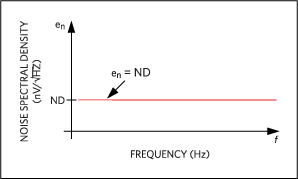Pink noise contains equal amounts of energy in each decade. It is characterized by a power spectral density (Figure 4) that is inversely proportional to frequency, thus the common name “1/f” noise.In Figure 4, Kv is a proportionally constant representing the extrapolated value of en at f = 1Hz. It is plotted on a log-log scale.

All noise found in semiconductor devices is a combination of white and pink noise, resulting in the noise spectral density curve shown in Figure 5, plotted on a log-log scale. The corner frequency (Fc) is the boundary between white and pink noise.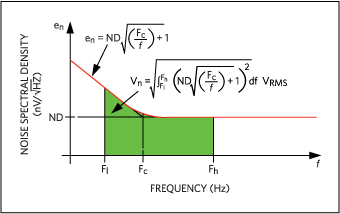The noise voltage present over any bandwidth is the area under the square of the noise spectral density curve, between the upper (Fh) and lower (Fl) frequencies of the band. Mathematically, this is written as:Simplifying:As can be seen, noise amplitude specifications must always be qualified by a frequency range.

### Types of Semiconductor Noise

As noted above, semiconductor noise sources can be placed in one of two categories depending on the shape of its spectral density curve. These categories are white and pink noise. We will take a closer look at each type.

#### White Noise

White noise is characterized by a uniform spectral density having equal energy in any given bandwidth. It is present in all active and passive devices. It gets its name from optics, where a broad spectrum light of constant amplitude appears white in color. White noise has the distinctive appearance on an oscilloscope as shown in Figure 6.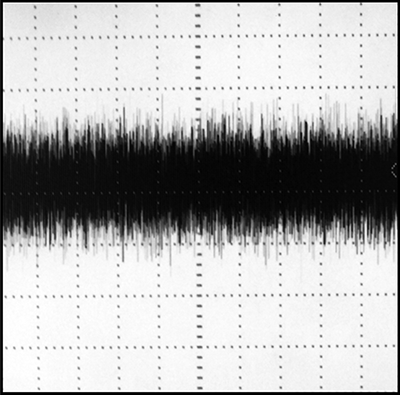The three sources of white noise in semiconductor devices are thermal, shot, and avalanche noise.

Thermal Noise

Thermal noise, also called Johnson noise, is present in all passive resistive elements and is caused by the random Brownian motion of electrons in the resistive medium. It increases with temperature and resistance and is often the greatest single source of semiconductor noise found in high-precision data converters.

All passive resistive elements generate thermal noise, whether discrete or integrated. Thermal noise increases with temperature and resistance, and the thermal noise level is unaffected by DC current. Resistors always generate noise, even in isolation.

Thermal noise density (ND) is defined as:

ND = √4kRT nV/√hZ

Where k is Boltzmann’s constant, R is resistance in Ohms, and T is the temperature in Kelvin.

Shot Noise

Shot noise, also called Schottky noise, is generated in active devices whenever a charge crosses a potential barrier found in transistors and diodes. This noise is generated because current flowing across a junction is not smooth, but is made of individual electrons arriving at random times. The DC current that we see at the macroscopic level is really the sum of many random microscopic pulses of current. This random variation in current flow is what gives shot noise its Gaussian white spectral density. Shot noise increases with current.

Shot noise density (ND) is defined as:

ND = √2qI na/√Hz

Where q is the electric charge and I is the current flowing through the barrier.

Avalanche Noise

Avalanche noise is found in PN junctions operating in reverse breakdown mode, such as zener diodes. The current generated during avalanche breakdown consists of randomly distributed noise spikes flowing through the reverse-biased junction. Like shot noise, avalanche noise requires the flow of current, but is usually much more intense.

#### Pink Noise

Pink noise is characterized by a spectral density that increases with decreasing frequency. It contains equal amounts of energy in each decade of bandwidth. This results in a power spectral density inversely proportional to frequency. Pink noise gets its name from optics, where a light spectrum dominated by lower frequencies appears pink in color. It is present in all active and some passive devices.

The two types of pink noise in semiconductor devices are flicker and popcorn noise.

Flicker Noise

Flicker noise (also called 1/f noise or contact noise) is excess noise generated by random fluctuations in current due to defects in semiconductor materials. It is found in all types of transistors and some types of resistors. Carbon composition and diffused resistors exhibit flicker noise because they are made with semiconductor material. Flicker noise is always associated with DC current.

Flicker noise density (ND) is defined as:

ND = K × I√1/f nA/√Hz

Where K is a device constant, I is the DC current, and f is the frequency.

Flicker noise is a category of noise phenomenon having various origins, for example:

1. In bipolar transistors it is caused by traps associated with contamination and crystal defects in the base-emitter junction.
2. In JFETs it is caused by carrier generation at traps in the channel depletion region.
3. In MOSFETs surface imperfections are the major culprit.

The frequency below which the flicker noise exceeds white noise is called the corner frequency (Fc). See Figure 5. Corner frequencies typically range between 0.1Hz and 1kHz, and will vary from source to source. All flicker noise sources add in RSS fashion and appear at the input or output of a semiconductor device as a single noise source having one net noise density and corner frequency.

Pink noise appears on an oscilloscope like white noise with an additional low frequency component. An example of pink noise is shown in Figure 7 below.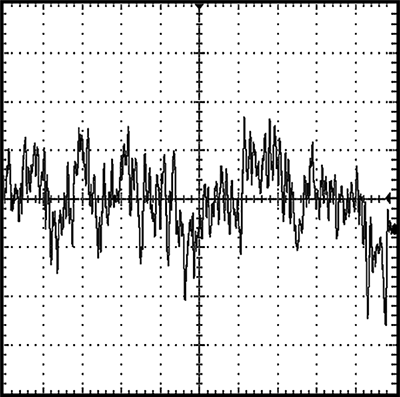Popcorn Noise

Popcorn noise (also called burst noise) is a low-frequency modulation of current caused by the capture and emission of charge carriers. It is most common in bipolar transistors and its cause is related to heavy-metal-ion contamination in semiconductor materials. It gets its name from the “popping” sound that it produces when played through a loudspeaker. The noise occurs randomly at rates below 100Hz, has a discrete amplitude, and a duration between 1ms and 1s.

Popcorn noise density (ND) increases with decreasing frequency.Where K is a device constant, I is the DC current, Fc is the corner frequency, and f is the frequency.

Popcorn noise appears on an oscilloscope as large, low-frequency, long-duration voltage steps. An example of popcorn noise is shown in Figure 8 below.All random noise sources add in RSS fashion; they appear at the input or output of an IC as a single noise source with one net noise density and corner frequency.

### How to Read Noise Specifications in a Data Sheet

Device noise specifications are located in either the Electrical Characteristics (EC) table or the Typical Operating Characteristics (TOC) section of a data sheet. A word of caution: noise is a “typical” specification and, therefore, not guaranteed. Noise specifications serve only to give a reasonable estimation of their values.

#### Time Domain Specifications

Data sheets usually specify noise in terms of voltage over a specified bandwidth, in volts peak-to-peak, and/or volts RMS. For example, Figure 9 shows how the MAX6129_21 voltage reference specifies noise voltage.The first noise specification listed, 30µVP-P, is the flicker or 1/f noise. It can be identified as flicker noise by the low-frequency band (0.1Hz to 10Hz) in the conditions column. Note that it is specified in µVP-P (rather than µVRMS) because noise over this band is of most interest in DC applications, where peak errors are a primary concern.

The second noise specification above is given in µVRMS. It is measured over a wider and higher frequency band of 10Hz to 1kHz, where white noise dominates and 1/f noise is negligible. Note that it is specified in µVRMS (rather than µVP-P) because noise specified over a wide band is of most interest in AC applications, where the signal-to-noise ratio (SNR) is of primary concern. Noise in SNR measurements are given in RMS. Noise voltage specifications will look very similar in ADC and DAC data sheets, as they do in voltage reference data sheets.

#### Frequency Domain Specifications

Noise may also be specified in terms of its spectral density (ND) at or near a specified spot frequency. For example, Figure 10 shows how the data sheet for the MAX6126_21 voltage reference specifies noise density.Because noise density always changes with frequency, it is specified at a particular frequency, called the spot frequency. The spot frequency is specified in the Conditions section of the EC table. In the MAX6126 the noise density is specified as 60nV/√Hz at a spot frequency of 1kHz. Other common spot frequencies include 10kHz, 100kHz, and 1MHz. Noise voltage, over any band, can be estimated using the spectral noise density (ND) from the EC table and the upper (Fh) and lower (Fl) frequencies of operation:

Vn = ND√(Fh - Fl VRMS

Equation 8 does not include flicker noise and, therefore, is valid only for frequency bands well above the corner frequency (Fl » Fc).

Noise voltage can, however, be predicted over any desired frequency band if the noise spectral density (ND) and corner frequency (Fc) are given. These specifications can be found in a device’s EC table or in a graph of the Noise Spectral Density over Frequency in the TOC section of the data sheet.

For example, the MAX6143 voltage reference contains the following noise spectral density plot (Figure 11) found in the TOC section of its data sheet.#### Estimating Noise Amplitudes Not Specified in an EC Table

If the spectral noise density (ND) and the corner frequency (Fc) are known, then the noise voltage over any frequency band can be estimated using Equations 1 and 3 repeated here:

VnP-P = 6.6 × VnRMSFour pieces of information are needed to estimate noise voltage:

1. ND, noise spectral density
2. Fc, corner frequency
3. Fl, lower frequency of the frequency band
4. Fh, upper frequency of the frequency band

ND and Fc can usually be found in either the EC table or from a noise spectral density plot found in the TOC section of the data sheet. The MAX6143 voltage reference will be used as an example. The flicker noise and the output noise voltage over audio bandwidth (20Hz to 20kHz) will be estimated. The circuit is shown below in Figure 12.Using the noise spectral density plot, Fc can be found at the intersection of the ND line and the 1/f line when plotted on a log-log scale. These lines are red in Figure 13.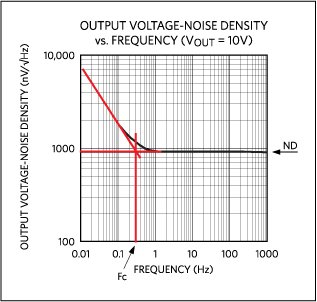In this case ND is 910nV/√Hz and Fc is 0.3Hz.

We use Equation 3 with the following values: ND = 910nV/√Hz, Fc = 0.3Hz, Fl = 0.1Hz, and Fh = 10Hz. The resulting noise voltage is 3.06µVRMS. Converting to VP-P by using Equation 1, we find that the flicker noise is 20.2µVP-P (Figure 14).These results agree closely with the data sheet value of 18µVP-P.

#### Finding a Noise Voltage Not Specified in the EC Table

Equations 1 and 3 can be used to find the output noise voltage over any frequency band of interest. For example, the noise voltage at the output of the MAX6143 can be found over the audio band of Fl = 20Hz to Fh = 20,000Hz.

Using the parameter values ND = 910nV/√Hz, Fc = 0.3Hz, Fl = 20Hz, and Fh = 20kHz, the noise voltage is calculated to be 128µVRMS (Figure 15).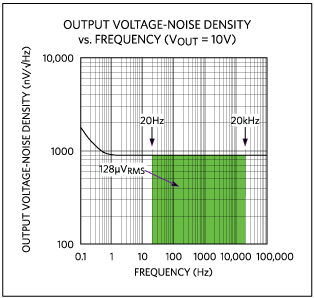### Thermal Noise Calculator

A free calculator is available for making quick work of these noise calculations. It can be downloaded. Click on the link and select the Thermal Noise Calculator (TNC). The TNC includes a User’s Guide that has instructions for the calculator's use, the theory and equations behind the issue, and most importantly, a practical example of its use in circuit design and analysis.

The TNC is a program written for the HP® 50g calculator that aids in the analysis of thermal noise found in resistors and other noise sources. This calculator finds the noise voltage generated by any device, if its white noise spectral density and 1/f corner frequency are known. Each parameter can be entered or found. The TNC can also be run on a Windows® PC using the free program HPUserEdit 5.4 found at www.hpcalc.org, or our calculator page.

Seven parameters (Figure 16) presented in this article can be entered or found:

1. Noise voltage (Vn) in µVP-P or µVRMS
2. White-noise spectral density (ND) in nV/√Hz
3. Johnson resistance (R) in Ω
4. Temperature (T) in °C
5. Upper frequency (Fh) in Hz
6. Lower frequency (Fl) in Hz
7. 1/f corner frequency (Fc) in Hz### Conclusion

All semiconductor devices generate noise from internal sources. All noise is unwelcome and designers must know how, or if, it can be reduced or eliminated. In this article we discussed the characteristics of the semiconductor noise and explained how it is specified in device data sheets. We demonstrated how to estimate the noise of a voltage reference under real-world conditions not specified in the data sheet.

In Part 2 of this series on managing noise in the signal chain we will focus on the sources of noise and distortion particular to data converters. We will also show how those noise sources are specified in a data sheet. We conclude in Part 3 by bringing together Parts 1 and 2 and show readers how to optimize their noise budget and choose the most appropriate data converter for their application.

### References

Lundberg, Kent, Noise sources in bulk CMOS, Massachusetts Institute of Technology, 77 Massachusetts Avenue, Cambridge, 2002.

Milotti, Edoardo, (2002) A pedagogical review of 1/f noise, Arxiv preprint physics/0204033, 2002.

Franco, Serghio, Design with Operational Amplifiers & Analog Integrated Circuits, McGraw-Hill Inc., New York, 1988.

Gray, Paul and Meyer, Robert. Analysis and Design of Analog Integrated Circuits, Wiley, New York, 1993.

Analog Devices Tutorial 719, “Understanding Voltage-Reference Topologies and Specifications.”